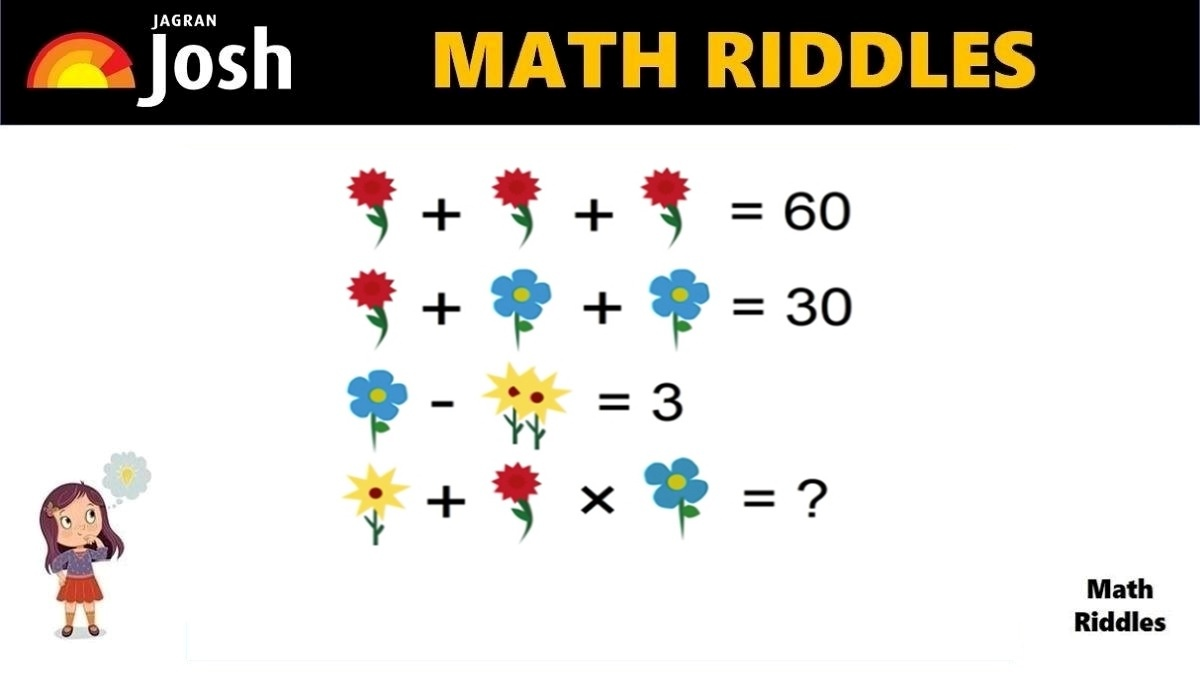# Can You Solve This Tricky Flower Math Riddle In 20 Seconds?

Math Riddles With Answers: Are you a math genius? Only 1% with a high IQ can solve this tricky math puzzle in 20 seconds.Can You Solve This Tricky Flower Math Riddle In 20 Seconds?

Try to solve this Math Puzzle within 20 seconds. If you enjoy solving riddles and math problems, then this math puzzle will be a treat for you. Difficult math puzzles are tricky more than regular math problems. These are usually represented with visual elements to make them impossible to solve. One has to have knowledge of mathematical concepts to be able to perform arithmetical operations correctly. We challenge Math enthusiasts to solve this tricky flower math problem.

If you love solving cryptic math puzzles, give this math puzzle a try! You have 20 seconds to find the correct answer.

## Math Riddles with Answers: Solution

Explanation

Row 1:  There are three red flowers which equal to 60.

So, we have sum of 3 red flowers = 60, if we divide both the LHS and RHS by 3 then we get the result that the value of 1 red flower is equal to 20. Now, we shall use the value of 1 red flower to solve the next row of the math problem. See the picture below to understand better.

Math Riddles With Answers: Can You Find The Value Of Lemon In 20 Seconds?

Row 2: Now, we have 1 red flower + 1 blue flower + 1 blue flower = 30.

We know that 1 red flower equals 20, so we apply the value and solve the equation to find the value of 1 blue flower. See the picture below to understand better.

So, now we know the value of 1 blue flower is 10. Let us find out the value of Yellow flower.

Genius Alert! Can You Find The Hidden Fish In This Picture Puzzle In 11 Seconds?

Row 3: Now, we have 1 blue flower – 2 yellow flowers = 3.

We already know that the value of 1 blue flower is 10. Hence, we apply the value of 1 blue flower to the equation to find the value of yellow flowers. See the picture below for better understanding.

Math Riddles With Answers: Can You Find The Value of Each Candy in 20 Seconds?

Row 4: Now, we have 1 yellow flower + 1 red flower x 1 blue flower = ?

One should not be quick to apply the values immediately. Notice carefully, the blue flower has only 4 petals in this equation. Hence, we shall take the value of 1 blue flower in this equation as 4. Look at the solved equation below.

Great Work!

## Tell us in comments: Did you solve this math puzzle correctly in 20 seconds?

Check out more amazing tricky math puzzles!

Math Riddles With Answers: Can You Solve This Tricky Math Rebus Puzzle 20 Seconds?

Math Riddles With Answers: Can You Find The Value of Burger, Fries, Coke in 20 Seconds?

Math Riddles With Answers: Can You Find The Value of Each Fruit in 20 Seconds?

Picture Puzzle: Find Two Same Emojis In 11 Seconds, 99% People Failed To Find Them

Picture Puzzle: Only 1% Genius Can Find The Hidden Words In The Picture in 11 seconds

Get the latest General Knowledge and Current Affairs from all over India and world for all competitive exams.
खेलें हर किस्म के रोमांच से भरपूर गेम्स सिर्फ़ जागरण प्ले पर